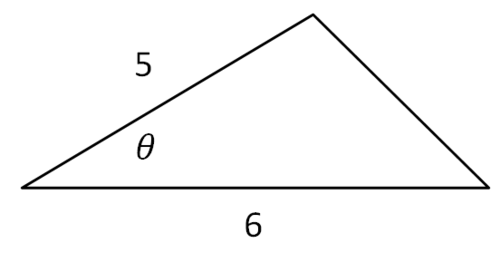# 4.7: Area of a Triangle

$$\newcommand{\vecs}{\overset { \rightharpoonup} {\mathbf{#1}} }$$ $$\newcommand{\vecd}{\overset{-\!-\!\rightharpoonup}{\vphantom{a}\smash {#1}}}$$$$\newcommand{\id}{\mathrm{id}}$$ $$\newcommand{\Span}{\mathrm{span}}$$ $$\newcommand{\kernel}{\mathrm{null}\,}$$ $$\newcommand{\range}{\mathrm{range}\,}$$ $$\newcommand{\RealPart}{\mathrm{Re}}$$ $$\newcommand{\ImaginaryPart}{\mathrm{Im}}$$ $$\newcommand{\Argument}{\mathrm{Arg}}$$ $$\newcommand{\norm}{\| #1 \|}$$ $$\newcommand{\inner}{\langle #1, #2 \rangle}$$ $$\newcommand{\Span}{\mathrm{span}}$$ $$\newcommand{\id}{\mathrm{id}}$$ $$\newcommand{\Span}{\mathrm{span}}$$ $$\newcommand{\kernel}{\mathrm{null}\,}$$ $$\newcommand{\range}{\mathrm{range}\,}$$ $$\newcommand{\RealPart}{\mathrm{Re}}$$ $$\newcommand{\ImaginaryPart}{\mathrm{Im}}$$ $$\newcommand{\Argument}{\mathrm{Arg}}$$ $$\newcommand{\norm}{\| #1 \|}$$ $$\newcommand{\inner}{\langle #1, #2 \rangle}$$ $$\newcommand{\Span}{\mathrm{span}}$$$$\newcommand{\AA}{\unicode[.8,0]{x212B}}$$

From geometry you already know that the area of a triangle is $$\frac{1}{2} \cdot b \cdot h$$

What if you are given the sides of a triangle are 5 and 6 and the angle between the sides is $$\frac{\pi}{3} ?$$ You are not directly given the height, but can you still figure out the area of the triangle?

## Finding the Area of Triangles

The sine function allows you to find the height of any triangle and substitute that value into the familiar triangle area formula.Using the sine function, you can isolate $$h$$ for height:

\begin{aligned} \sin C &=\frac{h}{a} \\ a \sin C &=h \end{aligned}

Substituting into the area formula:

\begin{aligned} \text {Area} &=\frac{1}{2} b \cdot h \\ \text {Area} &=\frac{1}{2} b \cdot a \cdot \sin C \\ \text {Area} &=\frac{1}{2} \cdot a \cdot b \cdot \sin C \end{aligned}

Recall that the variables used to note the sides and corresponding angles are arbitrary, so long as a side and its opposing angle share the same variable (side $$c$$ has an opposing angle $$C$$ ). If you were given $$\Delta A B C$$ with $$A=22^{\circ}, b=6, c=7$$ and asked to find the area, you would use the formula:

\begin{aligned} \text {Area} &=\frac{1}{2} b c \sin A \\ \text {Area} &=\frac{1}{2} \cdot 6 \cdot 7 \cdot \sin 22^{\circ} \approx 7.86 \text { units}^{2} \end{aligned}

The important part is that neither given side corresponds to the given angle.

Bonus Video: There is another way of finding the area of a triangle, Heron's Formula. This will be discussed in Example 5. Heron's formula is used when three side lengths are given.

## Examples

### Example 1

Earlier, you were given the sides of a triangle are 5 and 6 and the angle between the sides is $$\theta=\frac{\pi}{3}$$ and asked to find the area.Area $$=\frac{1}{2} \cdot 5 \cdot 6 \cdot \sin \frac{\pi}{3} \approx 12.99$$ units $$^{2}$$

### Example 2

Given $$\Delta X Y Z$$ has area 28 square inches, what is the angle included between side length 8 and $$9 ?$$

\begin{aligned} A r e a &=\frac{1}{2} \cdot a \cdot b \cdot \sin C \\ 28 &=\frac{1}{2} \cdot 8 \cdot 9 \cdot \sin C \\ \sin C &=\frac{28 \cdot 2}{8 \cdot 9} \\ C &=\sin ^{-1}\left(\frac{28 \cdot 2}{8 \cdot 9}\right) \approx 51.06^{\circ} \end{aligned}

### Example 3

Given triangle $$A B C$$ with $$A=12^{\circ}, b=4$$ and $$A r e a=1.7$$ units$$^{2},$$ what is the length of side $$c ?$$

\begin{aligned} \text {Area} &=\frac{1}{2} \cdot c \cdot b \cdot \sin A \\ 1.7 &=\frac{1}{2} \cdot c \cdot 4 \cdot \sin 12^{\circ} \\ c &=\frac{1.7 \cdot 2}{4 \cdot \sin 12^{\circ}} \approx 4.09 \end{aligned}

### Example 4

The area of a triangle is 3 square units. Two sides of the triangle are 4 units and 5 units. What is the measure of their included angle?

$$3=\frac{1}{2} \cdot 4 \cdot 5 \cdot \sin \theta$$
$$\theta=\sin ^{-1}\left(\frac{3 \cdot 2}{4 \cdot 5}\right) \approx 17.46^{\circ}$$

### Example 5

What is the area of $$\Delta X Y Z$$ with $$x=11, y=12, z=13 ?$$

Since none of the angles are given, there are two possible solution paths. You could use the Law of Cosines to find one angle.

Area $$=\frac{1}{2} \cdot 12 \cdot 13 \cdot \sin 52.02 \approx 61.5$$ units $$^{2}$$

The angle opposite the side of length 11 is approximately $$52.02^{\circ}$$ therefore the area is:

Area $$=\frac{1}{2} \cdot 12 \cdot 13 \cdot \sin 52.02 \approx 61.5$$ units $$^{2}$$

Another way to find the area is through the use of Heron's Formula which is:

Area$$=\sqrt{s(s-a)(s-b)(s-c)}$$

Where $$s$$ is the semiperimeter:

$$s=\frac{a+b+c}{2}$$

Using Heron's formula to find the area of ΔXYZ returns the same value:

$$s=\frac{a+b+c}{2}$$
$$s=\frac{11+12+13}{2}=\frac{36}{2}=18$$
$$A=\sqrt{18(18-11)(18-12)(18-13)}$$
$$A=\sqrt{18 \cdot 7 \cdot 6 \cdot 5}$$
$$A=\sqrt{3780} \approx 61.5$$ units $$^{2}$$

### Example 6

The area of a triangle is 3 square units. Two sides of the triangle are 4 units and 5 units. What is the measure of their included angle?

$$3=\frac{1}{2} \cdot 4 \cdot 5 \cdot \sin \theta$$
$$\theta=\sin ^{-1}\left(\frac{3 \cdot 2}{4 \cdot 5}\right) \approx 17.45 \ldots$$

Review

For $$1-11$$, find the area of each triangle.

1. $$\Delta A B C$$ if $$a=13, b=15$$, and $$\angle C=70^{\circ}$$.

2. $$\Delta A B C$$ if $$b=8, c=4,$$ and $$\angle A=58^{\circ}$$.

3. $$\Delta A B C$$ if $$b=34, c=29$$, and $$\angle A=125^{\circ}$$.

4. $$\Delta A B C$$ if $$a=3, b=7,$$ and $$\angle C=81^{\circ}$$.

5. $$\Delta A B C$$ if $$a=4.8, c=3.7,$$ and $$\angle B=54^{\circ} .$$

6. $$\Delta A B C$$ if $$a=12, b=5$$, and $$\angle C=22^{\circ}$$.

7. $$\Delta A B C$$ if $$a=3, b=10$$, and $$\angle C=65^{\circ}$$.

8. $$\Delta A B C$$ if $$a=5, b=9,$$ and $$\angle C=11^{\circ}$$.

9. $$\Delta A B C$$ if $$a=5, b=7,$$ and $$c=8$$.

10. $$\Delta A B C$$ if $$a=7, b=8,$$ and $$c=14$$.

11. $$\Delta A B C$$ if $$a=12, b=14,$$ and $$c=13$$.

12. The area of a triangle is 12 square units. Two sides of the triangle are 8 units and 4 units. What is the measure of their included angle?

13. The area of a triangle is 23 square units. Two sides of the triangle are 14 units and 5 units. What is the measure of their included angle?

14. Given $$\Delta D E F$$ has area 32 square inches, what is the angle included between side length 9 and $$10 ?$$

15. Given $$\Delta G H I$$ has area 15 square inches, what is the angle included between side length 7 and $$11 ?$$

4.7: Area of a Triangle is shared under a CC BY-NC license and was authored, remixed, and/or curated by LibreTexts.